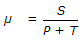# Civil Engineering - Highway Engineering

### Exercise :: Highway Engineering - Section 2

6.

On concrete roads, the camber generally provided, is

 A. 1 in 20 to 1 in 24 B. 1 in 30 to 1 in 48 C. 1 in 36 to 1 in 48 D. 1 in 48 to 1 in 60 E. 1 in 60 to 1 in 72

Explanation:

No answer description available for this question. Let us discuss.

7.

While calculating the sight distances, the driver's eye above road surface, is assumed

 A. 90 cm B. 100 cm C. 110 cm D. 120 cm E. 150 cm

Explanation:

No answer description available for this question. Let us discuss.

8.

If the number of lanes on the carriageway of a road is more than two, the total width of lane ways is equal to 3.0 m

 A. + 0.60 m B. + 0.70 m C. + 0.80 xn D. + 0.90 m E. + 1.50 m

Explanation:

No answer description available for this question. Let us discuss.

9.

Minimum thickness of a layer of fine sand required to cut off capillary rise of water completely, should be

 A. 40 cm B. 52 cm C. 64 cm D. 76 cm E. 80 cm

Explanation:

No answer description available for this question. Let us discuss.

10.

If W is the weight of a vehicle negotiating an upgrade 1 : S along a track having co-efficient of resistance μ, the tractive force T is given by

 A.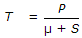B.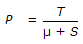C.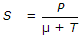D.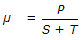E.